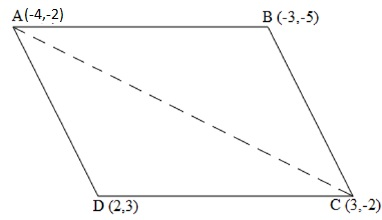Newbie

# Find the area of the quadrilateral whose vertices, taken in order, are (-4, -2), (-3, -5), (3, -2) and (2, 3). Q.4

• -1

what is the best solution for coordinate geometry of exercise 7.3. please help me to find the best and easy way to solve the coordinate geometry with tick.Find the area of the quadrilateral whose vertices, taken in order, are (-4, -2), (-3, -5), (3, -2) and (2, 3)

Share

1. Let the vertices of the quadrilateral be A (- 4, – 2), B ( – 3, – 5), C (3, – 2), and D (2, 3).

Join AC and divide the quadrilateral into two triangles.We have two triangles ΔABC and ΔACD.

Area of a triangle = 1/2 × [x1(y2 – y3) + x2(y3 – y1) + x3(y1 – y2)]

Area of ΔABC = 1/2 [(-4) {(-5) – (-2)} + (-3) {(-2) – (-2)} + 3 {(-2) – (-5)}]

= 1/2 (12 + 0 + 9)

= 21/2 square units

Area of ΔACD = 1/2 [(-4) {(-2) – (3)} + 3{(3) – (-2)} + 2 {(-2) – (-2)}]

= 1/2 (20 + 15 + 0)

= 35/2 square units

Area of quadrilateral ABCD = Area of ΔABC + Area of ΔACD

= (21/2 + 35/2) square units = 28 square units

• 1